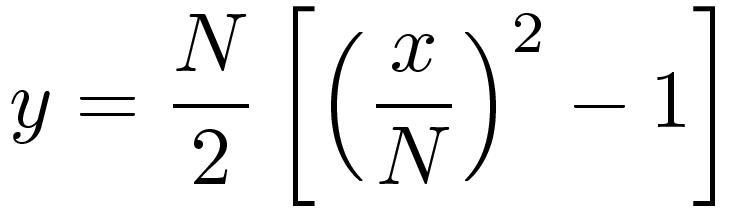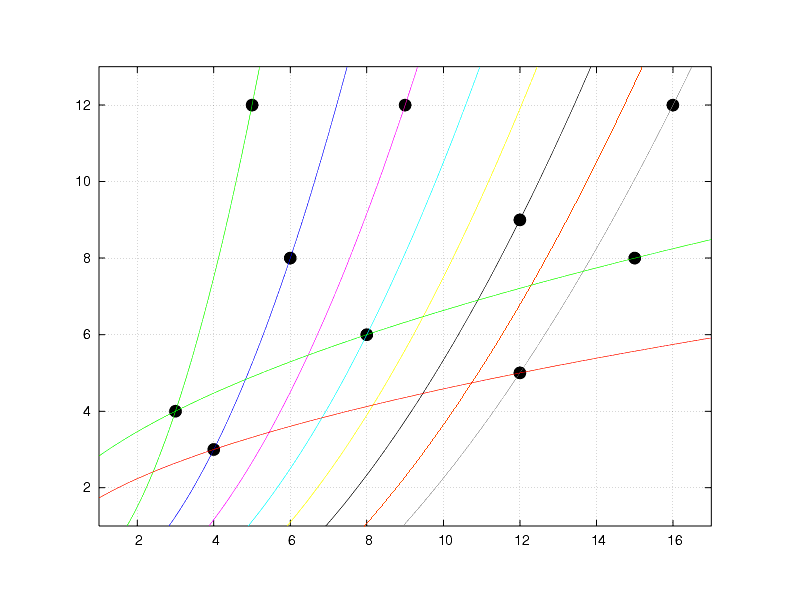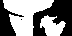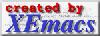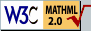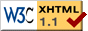## Rectangle Triangles (using MathML)

 ${4}^{2}+{3}^{2}={5}^{2}$ ${3}^{2}+{4}^{2}={5}^{2}$ ${36,87}^{o}$ ${5}^{2}+{12}^{2}={13}^{2}$ ${22,62}^{o}$ ${8}^{2}+{15}^{2}={17}^{2}$ ${28,07}^{o}$ ${7}^{2}+{24}^{2}={25}^{2}$ ${16,26}^{o}$ ${9}^{2}+{40}^{2}={41}^{2}$ ${12,68}^{o}$ ${12}^{2}+{35}^{2}={37}^{2}$ ${18,92}^{o}$ ${11}^{2}+{60}^{2}={61}^{2}$ ${10,39}^{o}$

## Trigonometric Functions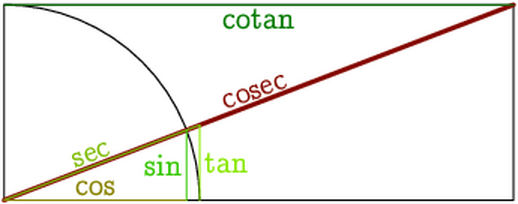## More Rectangle Triangles (without MathML)

 (3+2i)2+[2(i+1)(i+2)]2 =[1+2(i+1)(i+2)]2 132+842=852 152+1122=1132 (4+4i)2+[(2i+1)(2i+3)]2 =[2+(2i+1)(2i+3)]2 162+632=652 202+992=1012 Nothing new here: 92+122=152 152+362=392 212+722=752 272+1202=1232